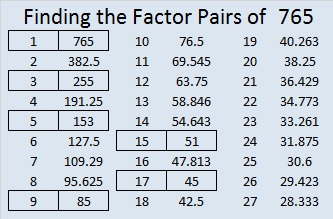# Simplifiable Square Roots up to √765

• 765 is a composite number.
• Prime factorization: 765 = 3 x 3 x 5 x 17, which can be written 765 = (3^2) x 5 x 17
• The exponents in the prime factorization are 2, 1, and 1. Adding one to each and multiplying we get (2 + 1)(1 + 1)(1 + 1) = 3 x 2 x 2 = 12. Therefore 765 has exactly 12 factors.
• Factors of 765: 1, 3, 5, 9, 15, 17, 45, 51, 85, 153, 255, 765
• Factor pairs: 765 = 1 x 765, 3 x 255, 5 x 153, 9 x 85, 15 x 51, or 17 x 45
• Taking the factor pair with the largest square number factor, we get √765 = (√9)(√85) = 3√85 ≈ 27.658633.765 is the 300th number whose square root can be simplified! Here are three tables with 100 simplifiable square roots each showing all the simplifiable square roots up to √765. When three or more consecutive numbers have simplifiable square roots, I highlighted them.That’s 300 simplifiable square roots found for the first 765 counting numbers. 300 ÷ 765 ≈ 0.392, so 39.2% of the numbers so far have simplifiable square roots.

Today’s puzzle is a whole lot less complicated than all that, so give it a try!Print the puzzles or type the solution on this excel file: 10 Factors 2016-02-04

Logical steps to find the solution are in a table at the bottom of the post.

—————————————

Here are some other fun facts about the number 765:

765 is made from three consecutive numbers so it is divisible by 3. The middle of those numbers is 6 so 765 is also divisible by 9.

765 can be written as the sum of two squares two different ways:

• 27² + 6² = 765
• 21² + 18² = 765

Its other two prime factors, 5 and 17, have a remainder of 1 when divided by 4 so 765² can be written as the sum of two squares FOUR different ways, two of which contain other numbers that use the same digits as 765. Also notice that 9 is a factor of each number in the corresponding Pythagorean triples.

• 117² + 756² = 765²
• 324² + 693² = 765²
• 360² + 675² = 765²
• 459² + 612² = 765²

765 can also be written as the sum of three squares four different ways:

• 26² + 8² + 5² = 765
• 22² + 16² + 5² = 765
• 20² + 19² + 2² = 765
• 20² + 14² + 13² = 765

765 is a palindrome in two different bases:

• 1011111101 BASE 2; note that 1(512) + 0(256) + 1(128) + 1(64) + 1(32) + 1(16) + 1(8) + 1(4) + 0(2) + 1(1) = 765.
• 636 BASE 11; note that 6(121) + 3(11) + 6(1) = 765.

—————————————